## AC Circuits

7-21-00

Sections 21.12 - 21.13

### Alternating current

Direct current (DC) circuits involve current flowing in one direction. In alternating current (AC) circuits, instead of a constant voltage supplied by a battery, the voltage oscillates in a sine wave pattern, varying with time as: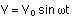In a household circuit, the frequency is 60 Hz. The angular frequency is related to the frequency, f, by: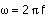Vo represents the maximum voltage, which in a household circuit in North America is about 170 volts. We talk of a household voltage of 120 volts, though; this number is a kind of average value of the voltage. The particular averaging method used is something called root mean square (square the voltage to make everything positive, find the average, take the square root), or rms. Voltages and currents for AC circuits are generally expressed as rms values. For a sine wave, the relationship between the peak and the rms average is:

rms value = 0.707 peak value

### Resistance in an AC circuit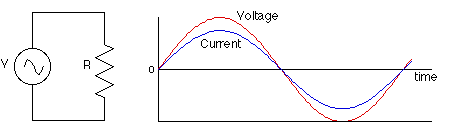The relationship V = IR applies for resistors in an AC circuit, soIn AC circuits we'll talk a lot about the phase of the current relative to the voltage. In a circuit which only involves resistors, the current and voltage are in phase with each other, which means that the peak voltage is reached at the same instant as peak current. In circuits which have capacitors and inductors (coils) the phase relationships will be quite different.

### Capacitance in an AC circuitConsider now a circuit which has only a capacitor and an AC power source (such as a wall outlet). A capacitor is a device for storing charging. It turns out that there is a 90° phase difference between the current and voltage, with the current reaching its peak 90° (1/4 cycle) before the voltage reaches its peak. Put another way, the current leads the voltage by 90° in a purely capacitive circuit.

To understand why this is, we should review some of the relevant equations, including:

relationship between voltage and charge for a capacitor: CV = Q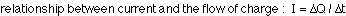The AC power supply produces an oscillating voltage. We should follow the circuit through one cycle of the voltage to figure out what happens to the current.

Step 1 - At point a (see diagram) the voltage is zero and the capacitor is uncharged. Initially, the voltage increases quickly. The voltage across the capacitor matches the power supply voltage, so the current is large to build up charge on the capacitor plates. The closer the voltage gets to its peak, the slower it changes, meaning less current has to flow. When the voltage reaches a peak at point b, the capacitor is fully charged and the current is momentarily zero.

Step 2 - After reaching a peak, the voltage starts dropping. The capacitor must discharge now, so the current reverses direction. When the voltage passes through zero at point c, it's changing quite rapidly; to match this voltage the current must be large and negative.

Step 3 - Between points c and d, the voltage is negative. Charge builds up again on the capacitor plates, but the polarity is opposite to what it was in step one. Again the current is negative, and as the voltage reaches its negative peak at point d the current drops to zero.

Step 4 - After point d, the voltage heads toward zero and the capacitor must discharge. When the voltage reaches zero it's gone through a full cycle so it's back to point a again to repeat the cycle.

The larger the capacitance of the capacitor, the more charge has to flow to build up a particular voltage on the plates, and the higher the current will be. The higher the frequency of the voltage, the shorter the time available to change the voltage, so the larger the current has to be. The current, then, increases as the capacitance increases and as the frequency increases.

Usually this is thought of in terms of the effective resistance of the capacitor, which is known as the capacitive reactance, measured in ohms. There is an inverse relationship between current and resistance, so the capacitive reactance is inversely proportional to the capacitance and the frequency:

A capacitor in an AC circuit exhibits a kind of resistance called capacitive reactance, measured in ohms. This depends on the frequency of the AC voltage, and is given by: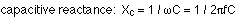We can use this like a resistance (because, really, it is a resistance) in an equation of the form V = IR to get the voltage across the capacitor: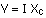Note that V and I are generally the rms values of the voltage and current.

### Inductance in an AC circuit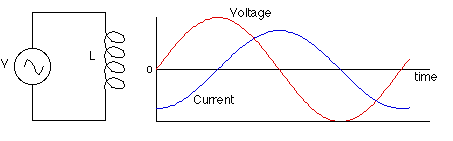An inductor is simply a coil of wire (often wrapped around a piece of ferromagnet). If we now look at a circuit composed only of an inductor and an AC power source, we will again find that there is a 90° phase difference between the voltage and the current in the inductor. This time, however, the current lags the voltage by 90°, so it reaches its peak 1/4 cycle after the voltage peaks.

The reason for this has to do with the law of induction: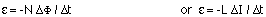Applying Kirchoff's loop rule to the circuit above gives: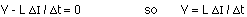As the voltage from the power source increases from zero, the voltage on the inductor matches it. With the capacitor, the voltage came from the charge stored on the capacitor plates (or, equivalently, from the electric field between the plates). With the inductor, the voltage comes from changing the flux through the coil, or, equivalently, changing the current through the coil, which changes the magnetic field in the coil.

To produce a large positive voltage, a large increase in current is required. When the voltage passes through zero, the current should stop changing just for an instant. When the voltage is large and negative, the current should be decreasing quickly. These conditions can all be satisfied by having the current vary like a negative cosine wave, when the voltage follows a sine wave.

How does the current through the inductor depend on the frequency and the inductance? If the frequency is raised, there is less time to change the voltage. If the time interval is reduced, the change in current is also reduced, so the current is lower. The current is also reduced if the inductance is increased.

As with the capacitor, this is usually put in terms of the effective resistance of the inductor. This effective resistance is known as the inductive reactance. This is given by:where L is the inductance of the coil (this depends on the geometry of the coil and whether its got a ferromagnetic core). The unit of inductance is the henry.

As with capacitive reactance, the voltage across the inductor is given by: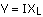### Where does the energy go?

One of the main differences between resistors, capacitors, and inductors in AC circuits is in what happens with the electrical energy. With resistors, power is simply dissipated as heat. In a capacitor, no energy is lost because the capacitor alternately stores charge and then gives it back again. In this case, energy is stored in the electric field between the capacitor plates. The amount of energy stored in a capacitor is given by: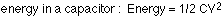In other words, there is energy associated with an electric field. In general, the energy density (energy per unit volume) in an electric field with no dielectric is: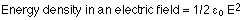With a dielectric, the energy density is multiplied by the dielectric constant.

There is also no energy lost in an inductor, because energy is alternately stored in the magnetic field and then given back to the circuit. The energy stored in an inductor is: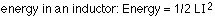Again, there is energy associated with the magnetic field. The energy density in a magnetic field is:### RLC Circuits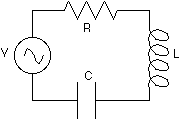Consider what happens when resistors, capacitors, and inductors are combined in one circuit. If all three components are present, the circuit is known as an RLC circuit (or LRC). If only two components are present, it's either an RC circuit, an RL circuit, or an LC circuit.

The overall resistance to the flow of current in an RLC circuit is known as the impedance, symbolized by Z. The impedance is found by combining the resistance, the capacitive reactance, and the inductive reactance. Unlike a simple series circuit with resistors, however, where the resistances are directly added, in an RLC circuit the resistance and reactances are added as vectors.

This is because of the phase relationships. In a circuit with just a resistor, voltage and current are in phase. With only a capacitor, current is 90° ahead of the voltage, and with just an inductor the reverse is true, the voltage leads the current by 90°. When all three components are combined into one circuit, there has to be some compromise.

To figure out the overall effective resistance, as well as to determine the phase between the voltage and current, the impedance is calculated like this. The resistance R is drawn along the +x-axis of an x-y coordinate system. The inductive reactance is at 90° to this, and is drawn along the +y-axis. The capacitive reactance is also at 90° to the resistance, and is 180° different from the inductive reactance, so it's drawn along the -y-axis. The impedance, Z, is the sum of these vectors, and is given by: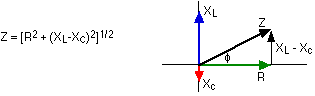The current and voltage in an RLC circuit are related by V = IZ. The phase relationship between the current and voltage can be found from the vector diagram: its the angle between the impedance, Z, and the resistance, R. The angle can be found from: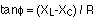If the angle is positive, the voltage leads the current by that angle. If the angle is negative, the voltage lags the currents.

The power dissipated in an RLC circuit is given by: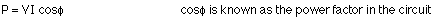Note that all of this power is lost in the resistor; the capacitor and inductor alternately store energy in electric and magnetic fields and then give that energy back to the circuit.